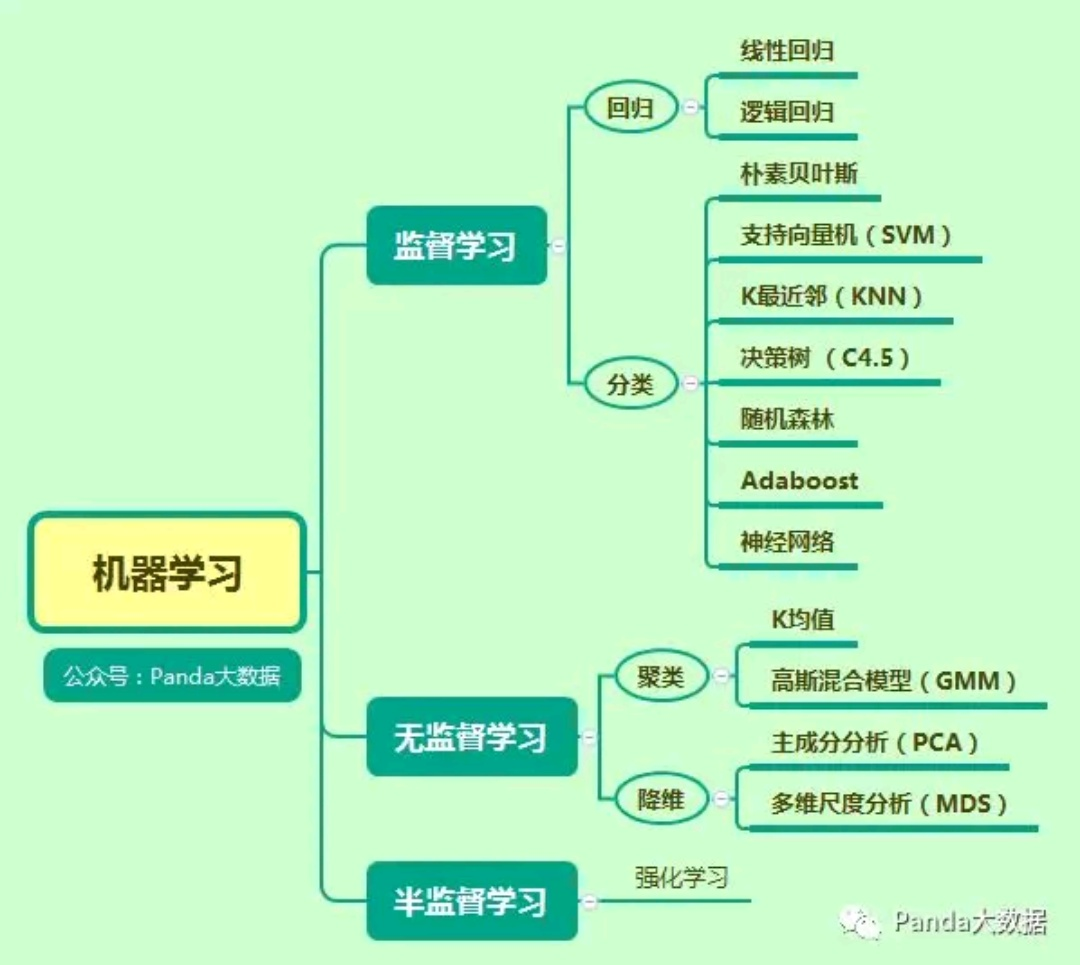# 机器学习（1.13）

## 机器学习-无监督学习

Machine Learning - Unsupervised Learning

• 定义
• 算法
• 小结

### 定义

#### 无监督学习

$\left({x}^{\left(1\right)},{y}^{\left(1\right)}\right),\left({x}^{\left(2\right)},{y}^{\left(2\right)}\right)$$(x^{(1)},y^{(1)}), (x^{(2)},y^{(2)})$

$\left({x}^{\left(1\right)}\right),\left({x}^{\left(2\right)}\right)$$(x^{(1)}), (x^{(2)})$

• EM算法
• K均值
• 高斯混合模型（GMM）
• 主成分分析（PCA）
• 多维尺度分析（MDS）
• 受限玻尔兹曼机

### 小结